All Exams Free Study Material and PDFs Join Our Telegram Channel Click Here Join Now

# Pipe & Cistern Short-Cut Tricks & Tips

Pipe & Cistern Short-Cut Tricks & Tips : Pipe & Cistern Short-Cut Tricks & Tips Question Pdf for Banking, SSC, RRB, FCI, Railway, UPSC, State PCS, Insurance & other Competitive exams. Pipe & Cistern Short-Cut Tricks & Tips shortcut Tricks Pdf, Pipe & Cistern Short-Cut Tricks & Tips MCQ, Pipe & Cistern Short-Cut Tricks & Tips Objective Question & Answer Pdf. “Pipe & Cistern Short-Cut Tricks & Tips Questions PDF” In this post we are providing you the Pipe & Cistern Short-Cut Tricks & Tips pdf with detailed solution & Short Tricks. So that you can easily get the logic of question. This Pipe & Cistern Short-Cut Tricks & Tips Pdf we are Providing is free to download. ” Most Important Pipe & Cistern Short-Cut Tricks & Tips Question PDF with Answers

Pipe & Cistern Short-Cut Tricks & Tips Plays a vital role in Exam. In every exam you will get at least 5-10 questions from this topic. So candidates must focus on this topic and download this Pipe & Cistern Short-Cut Tricks & Tips pdf to get important questions with best solution regarding Pipe & Cistern Short-Cut Tricks & Tips. We have put all Previous Year Questions of Pipe & Cistern Short-Cut Tricks & Tips that are Asked in various Govt & Private Exam.

#### Problems containing pipes and cisterns fall broadly into one of two categories. The first category being one wherein questions are concerned with the time required to fill up a tank, with a combination of one or more pipes and cisterns. The second category, concerns itself with leakages and holes, requiring you to calculate the effective rate of the tank being filled or emptied.

The same principle of Time and Work is employed to solve the problems on Pipes and Cisterns. The only difference is that in this case, the work done is in terms of filling or emptying a cistern (tank) and the time taken is the time taken by a pipe or a leak (crack) to fill or empty a cistern respectively.
Inlet : A pipe connected with a tank (or a cistern or a reservoir) is called an inlet, if it fills it.
Outlet : A pipe connected with a tank is called an outlet, if it empties it.

⇒ If a pipe can fill a tank in x hours, then the part filled in 1 hour =  1 / x
⇒ If a pipe can empty a tank in y hours, then the part of the full tank emptied in 1 hour=  1 /  y⇒If a pipe can fill a tank in x hours and another pipe can empty the full tank in y hours, then the net part filled in 1
hour, when both the pipes are opened  1/ x – 1 /yTime taken to fill the tank, when both the pipes are opened =  xy /  y-x⇒If a pipe can fills or empties tank in x hours and another can fill or empties the same tank in y hours, then time taken to fill or empty the tank xy / y + x , when both the pipes are opened
⇒If a pipe fills a tank in x hours and another fills the same tank is y hours, but a third one empties the full tank in z
hours, and all of them are opened together, then net part filled in 1 hr =1/x +1/y – 1/z⇒ A pipe can fill a tank in x hrs. Due to a leak in the bottom it is filled in y hrs. If the tank is full, the time taken by the leak to empty the tank = xy / y – x Hours.
⇒A cistern has a leak which can empty it in X hours. A pipe which admits Y litres of water per hour into the cistern is
turned on and now the cistern is emptied in Z hours. Then the capacity of the cistern is =  X+Y+Z / Z – X hours.

## CISTERN:

Cistern is referred to a tank/Reservoir which has some specific capacity to hold liquid.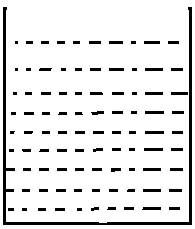## PIPES: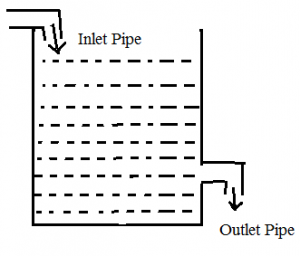There are two types of Pipes,They are

1)Inlet Pipe

2)Outlet Pipe

Inlet Pipe:

Inlet Pipe is used to fill the tank.

Outlet Pipe:

Outlet Pipe is used to empty the tank.

There may be any number of Inlet Pipes and Outlet Pipes that are connected to a cistern.

Before Getting into this topic,let us remember 3 basic terms

Total Capacity=LCM(Given Numbers)(unit-Litres)

Time=Total Capacity/Efficiency(unit-hr,sec,min,day)

Efficiency=Total Capacity/Time(unit Litre/hour)

## #.1.TYPE 1:

### PROBLEMS BASED ON INDIVIDUAL CAPACITY:

There are 3 sub type in this type,they are

1)When both the pipes are Inlet Pipes

2)When both the pipes are Outlet Pipes

3)One Pipe is Inlet Pipe and Outlet Pipe

1)When both the pipes are Inlet Pipes:

1)Pipe A can fill the tank in 10 hrs and Pipe B can fill the tank in 40 hrs.How long will it take to fill the tank if both the pipes are opened?

Explanation

Given that,

Pipe A can fill the tank in 10 hrs and Pipe B can fill the tank in 40 hrs

Total Capacity=LCM(10,40)=40Litres

A’s Efficiency=40/10=4 litre/hour

B’s Efficiency=40/40=1 litre/hour

So,

When both are opened,their total capacity=4+1=5 Litre/hour

Time=40/5=8 hours

So,When Pipe A and B are opened together,they can fill the tank in 8 hours

2)When both the pipes are Outlet Pipes:

1)Pipe A can empty the tank in 3 hours where as Pipe B can empty the tank in 6 hours.When they opened together how,long will it take to empty the tank?

Explanation

Given that,

Pipe A can empty the tank in 3 hours where as Pipe B can empty the tank in 6 hours.

Total Capacity of the tank=LCM(3,6)=6 Litres

A’s Efficiency=6/3=2litres/hour

B’s Efficiency=6/6=1 litre/hour

Total Efficiency of (A+B)=(2+1=3litres/hour)

Time=6/3=2hours

Therefore,When A & B are opened together,they can empty the tank in 2 hours

3)One Pipe is Inlet Pipe and Outlet Pipe:

1)A can fill the tank in 12 hours,B can empty the tank in 15 hours.When both the pipes are opened find how long will it take to empty/fill the tank ?

Explanation

Given that,

A can fill the tank in 12 hours,B can empty the tank in 15 hours

Total Capacity=LCM(12,15)=60 Litres

A’s(Inlet Pipe) Efficiency=60/12=5litre/hour

B’s(Outlet Pipe)Efficiency=60/15=4litre/hour

Note:

If the Efficiency of Inlet Pipe is greater then the Outlet Pipe the tank will be filled,

If the Efficiency of Outlet Pipe is greater then the Inlet Pipe then the tank will be Emptied

Inlet Pipe fill 5 litre in 1 hour at the same time Outlet Pipe empty 4 Litre in 1 hour so,in 1 hour only 1 litre is filled in the tank.

Time taken to fill the tank=60/1=60hours

## #.2.TYPE 2:

### BASED ON PARTIAL WORK:

1)Pipe A can fill the tank in 15 minutes and Pipe B can fill the tank in 20 Minutes.They opened together for 4 Minutes and after that B was turned off.How long will A take to fill the Remaining tank capacity?

Explanation

Given that,

Pipe A=15 minutes(fill)

Pipe B=20 minutes(fill)

Total Capacity=LCM(15,20)=60litres

Efficiency of A=60/15=4litre/min

Efficiency of B=60/20=3litre/min

They were Opened for 4 Minutes,

4+3=7litre/min

7*4=28 Litre.

Remaining 60-28=32 litre

After that B was turned off,then 32 litre is filled by Pipe A

=32/4=8 min

## #.3. TYPE 3:

### BASED ON EFFICIENCY:

1)A is thrice as efficient as B,If both the pipes are opened together the tank is filled in 2 days,then how long will it take to fill the tank only if Pipe B is Opened?

Explanation

Let us Assume the capacity of Pipe B=10litre/day

Therefore,Capacity of Pipe A=3(B)

=3(10)=30litre/day

So When they are opened together,they can fill 40 litres/day

In 2 days,they can fill 80litres

If Pipe B is Opened then it will take-80/10=8 days

## #.4. TYPE 4

### BASED ON TIME:

1)There are 4 inlet Pipes namely A,B,C,D and their individual capacity to fill the tank is 20 hours,30 hours,40 hours and 50 hours respectively.A opens at 6.00 A.M.,B opens at 8.00 A.M.,C opens at 9 A.M. and D opens at 10 A.M.Find at what time the tank will be filled?

Explanation

Total Capacity=LCM(20,30,40,50)=600 Litres

A’s Efficiency=600/20=30litres/hour

B’s Efficiency=600/30=20litres/hour

C’s Efficiency=600/40=15 litres/hour

D’s Efficiency =600/50=12 litres/hour

Given that,

A is opened at 6.00 A.M. till 10.00 A.M,it is opened for 4 hours

=30*4=120 litres/hour

B is opened at 8.00 A.M. till 10 A.M.,it is opened for 2 hours

=20*2=40 litres/hour

C is opened at 9.00 A.M. till 10 A.M., it is opened for 1 hour

=15*1=15 litres/hour

At 10.00 A.M

Three Pipes (A,B & C fill 175 litres)

Remaining Capacity in the tank=600-175=425 litres

This 475 litres is filled by all the 4 Inlet Pipes

Time=475/(30+20+15+12)

=475/77

=5 hours 30 Minutes

So from 10 A.M. it would take 5 hours 30 Minutes

The tank will be filled at 3.30 P.M

## #.5 TYPE 5:

### BASED ON PARTIAL TIME:

1)Two Pipes A & B fill the tank in 60 Minutes and 40 Minutes respectivley.How many minutes will it take to fill the tank from empty state if B is used for half the time & A and B used to fill Other half?

Explanation

Let the Total Capacity=LCM(60,40)=120 Litres

Efficiency of A=120/60=2 litre/minute

Efficiency of B=120/40=3 litre/minute

Let the time taken by the pipes to fill the tank be X

Half the time=X/2 is used to fill the tank by B & Another half the time is used to fill the tank by A+B

=X/2(Filled by Pipe B)+X/2(Filled by Pipe A+B)

=(X/2)* (3) + (X/2)* (5)

=3(X/2) + 5(X /2)

=8(X/2)

We know that

Total Capacity=Time * Efficiency

120 = (X/2) * 8

240=X*8

30=X

Therefore, the tank is filled in 30 Minutes

(Q1)Two pipes A and B can fill a tank in 20 minutes and 30 minutes respectively.If both pipes are opened together, the time taken to fill the tank is.

Solution: Fig.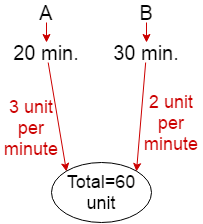Rate of Filling the tank by A and B in 1 minute=3+2=5 unit

So, total time taken by Pipe (A+B)=60/5

=12 minutes Ans.

[Concept: First step-L.C.M=20,30=60 i.e total unit. now 60/20=3 unit per minutes tank filling by pipe A and 60/30=2 unit per minutes tank filling by pipe B.]

(Q2) A tap can empty a tank in 30 minutes. A second tap can empty it in 45 minutes. If both the taps operate simultaneously, how much time is needed to empty the tank?

Solution: Fig.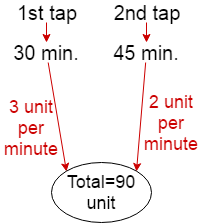Rate of emptying the tank by 1st and 2nd tap in 1 minute=(3+2)

=5 unit

So, total time taken by (1st+2nd)tap=90/5

=18 minutes Ans.

[Concept: First step-L.C.M=30,45=90 i.e total unit. now 90/30=3 unit per minutes tank emptying by 1st tap and 90/45=2 unit per minutes tank emptying by 2nd tap.]

(Q3)Three tapes A, B, C can fill an overhead tank in 4, 6 and 12 hours respectively. How long would the three taps take to fill the tank if all of them are opened together?

Solution: Fig.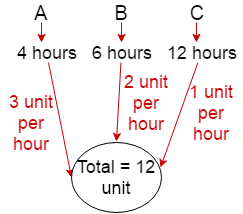Rate of Filling the tank by A, B and C in 1 hour=(3+2+1)

=6 unit

So, total time taken by (A+B+C)=12/6

=2 hours Ans.

(Q1)A water tank can filled by a tap in 30 minutes and another tap can fill it in 60 minutes. If both the taps are kept open for 5 minutes and then the first tap is closed, how much time 2nd tap will take to fill the remaining tank.

Solution: Fig.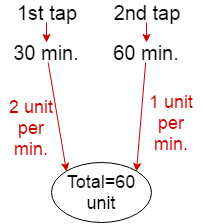Rate of Filling the tank by 1st tap and 2nd tap in 1 minute=(2+1)

=3 unit

[Concept: From fig.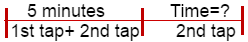According to question 1st tap and second tap को  5 minutes तक एक साथ खुला रखा गया है तो दोनों 5 minutes में कितना water fill करेगा वो निकाल लेंगे| 5 minutes के बाद तो 1st tap बंद कर दिया जा रहा है तो सिर्फ अब tank के remaining unit  को fill करने के लिए 2nd tap ही बचा है तो वो कितना time लेगा वो निकाल लेंगे. ]

Rate of Filling the tank by 1st tap and 2nd tap in 5 minute=5×3=15 unit

Remaining=60-15=45 unit that is filled by 2nd tap

So, total time taken by 2nd tap=45/1

=45 minutes Ans.

(Q2)Three pipes A, B and C can fill a tank in 6 hours, 9 hours and 12 hours respectively. B and C are opened for half an hour, then A is also opened. The time taken by the three pipes together to fill the remaining part of the tank is.

Solution: Fig.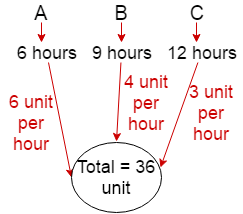Rate of Filling the tank by A+B+C in 1 hour=(6+4+3)unit

=13 unit

[ Concept: From fig.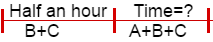half an hour तक B and C tank fill करेगा |half an hour के बाद A को open किया जाता है तो अब A, B and C मिलकर करेगा| ]

Rate of Filling the tank by B+C in 1 hour=(4+3)unit

=7 unit

Rate of Filling the tank by B+C in half an hour=7/2 unit

Remaining unit=36-(7/2)=65/2 unit

So, total time taken by (A+B+C)=(65/2)/13

=2 ½ hours Ans.

(Q3)Three pipes A, B and C can fill a tank in 6 hours. After working together for 2 hours, C is closed and A and B fill the tank in 8 hours. The time(in hours) in which the tank can be filled by pipe C alone is.

Solution:

Let tank fill by A+B+C in 1 hour= 1 unit

Tank fill by A+B+C in 6 hour= 6 unit

After working together for 2 hours then

Tank fill by A+B+C in 2 hour= 2 unit

Remaining = 6 – 2=4 unit that is filled by A and B because C is closed

Rate of filling of A+B in 1 hour=4/8=0.5 unit

Rate of filling of C in 1 hour=1-0.5=0.5 unit

Time taken by C = 6/0.5= 12 hours Ans.

## Common Type Of Pipe And Cistern Problem Shortcut Tricks

(Q1)A tank has two pipes. The first pipe can fill it in 4 hours and the second can empty it in 16 hours. If two pipes are opened together at a time, then the tank will be filled in.

Solution: Fig.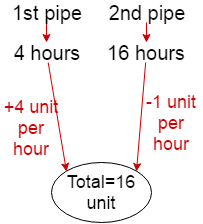Rate of filling the tank by 1st pipe= 4 unit per hour

Rate of emptying the tank by 2nd pipe= 1 unit per hour

Net rate of filling the tank=4-1=3unit

so required time=16/3=5 ¹/3 hours Ans.

[concept:जब एक pipe tank को fill(भर)कर रहा हो or दूसरा empty(खाली) कर रहा हो तो जो pipe tank को fill कर रहा होगा, तो उसका Rate of filling the tank (+ve) में लेना है or जो pipe tank को empty कर रहा होगा तो उसका Rate of emptying the tank   ( -ve) में लेना है| इसलिए figure में +ve or -ve लिया गया है.]

(Q2)Two pipes A and B can fill a cistern in 3 hours and 5 hours respectively. Pipe C can empty in 2 hours. If all the three pipes are open, in how many hours the cistern will be full?

Solution: Fig.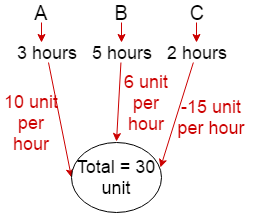Rate of filling a cistern by A= 10 unit per hour

Rate of filling a cistern by B= 6 unit per hour

Rate of emptying a cistern by C= -15 unit per hour

Net rate of filling the tank by (A+B-C)=10+6-15

=1 unit per hour

so total time taken by (A+B-C)=30/1

=30 hours Ans.

(Q1)Two pipes A and B can fill a tank with water in 30 minutes and 45 minutes respectively. Another pipe C can empty the tank in 36 minutes. First A and B are opened. After 12 minutes, C is also opened. The tank is filled up in.

Solution: Fig.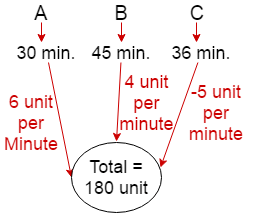[ Concept: From fig.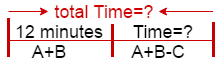]

Rate of filling the tank by (A+B) in 1 minute=(6+4)=10 unit

Rate of filling the tank by (A+B) in 12 minute=12×10=120 unit

Remaining=180-120=60 unit that is filled by A, B and C

Rate of emptying the tank by C = -5 unit per minute

Net rate of filling the tank by (A+B-C)=(6+4-5)

=5unit per minute

so required time taken by (A+B-C)=60/5

=12 minutes

Total time=12+12=24 minutes Ans.

## Practice Questions

### Ques 1.

Two pipes P and Q can fell a cistern in 24 min. and 36 min. respectively . Third pipe R can empty it in 36 min. If all the three pipes are opened , find the taken to fill the cistern.
(a) 1 hour
(b) 24 mins
(c) 36 mins
(d) 30 mins

### Ques 2.

A tank has a leak which would empty it in 8 hours. A tap is turned on which admit 6 liters a minute into the tank and it now emptied in 12 hours . How many literes does the tank holds?

(a) 8260 ltr
(b) 8640 ltr
(c) 8560 ltr
(d) 8800 ltr

### Ques 3.

When the waste pipe is closed , two taps can separately fill a cistern in 10 and 12 minutes respectively .when the waste pipe is opened they together fill it in 15 minutes . How long does it take waste pipe to empty the cistern , when the taps are closed?
(a) 8 min. 34 sec.
(b) 7 min.10 sec.
(c) 12 min.
(d) 10 min

### Ques 4.

Two pipes can fill a tank in 10 minutes and 30 minutes respectively and a third pipe can empty the full tank in 20 minutes . If all the three pipes are opened simultaneously, the tank will be filled in:
(a) 12 minutes
(b) 10 minutes
(c) 8 minutes
(d) 6 minutes

### Ques 5.

Two pipes can fill a cistern in 14 hours and 16 hours respectively . The pipes are opened simultaneously and it is found that due to leakage in the bottom , 32 minutes extra are taken for the cistern to be filled up. When the cistern is full , in what time will the leak empty it ?
(a) 108 hours
(b) 112 hours
(c) 116 hours
(d) 120 hours

### Ques 6.

A cistern can be filled by pipes A and B in 12 minutes and 10 minutes respectively . The full tank can be emptied by a third pipe C in 8 minutes only .If all the pipes be turned on at the same time,the cistern will be full in :
(a) 17 min
(b) 171/7 min.
(c) 17 2/7 min.
(d) 18 min.

### Ques 7.

If two pipes function simultaneously , the reservoir will be filled in 12 hours. One pipe fills the reservoir 10 hours faster than the other. How many hours does it take the second pipe to fill the reservoir?
(a) 20 hours
(b) 25 hours
(c) 30 hours
(d) 40 hours

### Ques 8.

A tank is filled in hours by three pipes A, B and C . The pipe C is twice as fast as B and B is twice as fast as A. How much time will pipe A alone take to fill the tank?
(a) 40 hours.
(b) 35 hours
(c) 30 hours
(d) 25 hours

### Ques 9.

Three pipes P , Q and R can fill a tank in 6 hours , After working it at together for 2 hours , R is closed and P and Q can fill the remaining part in 7 hours . How much time will R take alone to fill the tank?
(a) 16 hours
(b) 14 hours
(c) 12 hours
(d) 10 hours

### Ques 10.

Three taps P, Q and R can fill a tank in 12, 15 and 20 hours respectively . If P is opened all the time and Q and R are opened for one hour each alternately , the tank will be full in
(a) 5 hours
(b) 6 hours
(c) 6 2/3 hours
(d) 7 hours

### solutions:

Ans 1.Since time taken to fill the cistern by Q = time taken to empty the cistern by R so

the cistern will be filed by P in 24 min.

Ans 2. In I hour the part filled by the tap = 1/8 -1/12 = 1/24
Hence , the tap can fill the tank in 24 hours.
Therefore, capacity of the tank = 24 x 60 x 6 = 8640 litres.

Ans 3. The waste pipe can empty in 1 min .= 1/10 + 1/12 – 1/15 = 11/60 – 1/15 = 7/60
of the cistern.

Hence, the waste pipe can empty the cistern in 60/7 minutes , i.e., 8 minutes 34 sec.

Ans 4
. 1n I minute the part filled by all the three pipes = 1/10 + 1/30 – 1/20 = 1/12

Hence , all the three pipes will fill the bank in 12 minutes.

Ans 5. In I hour the part filled by both the pipes = 1/14 + 1/16 = 15/112
Hence, both the pipes will fill the cistern in 112/15 min.
but due to leakage , the tank will be filled in 112/15 + 32/60 = 120/15 = 8 hours.
Since, in 1 hr. the part emptied by the leakage = 15/112 – 1/8 = 1/112
Therefore , leakage will empty the tank in 112 hours.

Ans 6
. In 1 minute the part filled by the three pipes = 1/12 + 1/10 -1/8 = 7/120

Hence, all the three pipes will fill the cistern in 120/7 = 17 1/7 minutes.

Ans 7.Let ( x- 10) and x hrs be the time taken by two pipes to fill the reservoir , then,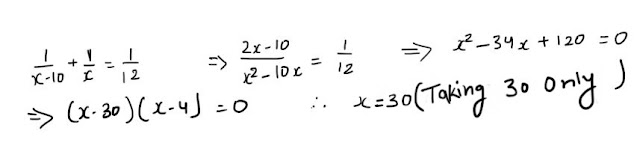Hence , second pipe will fill the reservoir in 30 hours.

Ans 8.
Let x,x/2 and x/4 hours be the time taken by pipes A, B and C respectively to

fill the tank,then
1/x + 2/x + 4/x = 1/5 => 7/x = 1/5            ∴ x = 35 hours.
Hence , time taken by A is 35 hours to fill the tank.Ans 9. In 2 hour the part filled by all the three pipes = 2 X 1/6 = 1/3
Remaining part = 1 – 1/3 = 2/3, which is filled by ( P + Q ) in 7 hours.
Hence , in 1 hour the part filled by ( P + Q ) = 2/ 3 x 7 = 2/21
Since , in 1 hour the part filled by R alone = 1/6 – 2/21= 1/14
Therefore, R alone will fill the bank in 14 hours.Ans 10.In 1 hour the part filled by (P + Q ) = 1/12 + 1/15 = 3/20
In 1 hour the part filled by (P + R ) = 1/12 + 1/20 = 2/15
Hence, in 2 hours the part filled by ( P+ Q +R ) = 3/20 + 2/15 = 17/60
Then , in 6 hours the part filled by ( P + Q + R ) = 3 x 17/60 = 17/20
Remaining part = 1 – 17/20 = 3/20 , which is filled by ( P + Q ) in 1 hour.
Hence, total time taken = 6 + 1 = 7 hours.

# Practice Problems on Pipes and Cistern

• A Special pump can be used for filling as well as for emptying a Cistern. The capacity of the Cistern is 2400m³. The emptying capacity of the Cistern is 10m³ per minute higher than its filling capacity and the pump needs 8 minutes lesser to Cistern the tank than it needs to fill it. What is the filling capacity of the pump?
A. 40m³/min
B. 50m³/min
C. 60m³/min
D. 30m³/min
E. None of the Above

Explanation :
Filling Capacity of the Pump = x m/min
Emptying Capacity of the pump = (x+10) m/min
2400/x – 2400/x+10 = 8
(x – 50) + (x + 60) = 0
x = 50
• Three pipes P, Q and R can fill a Cistern in 6 hours. After working at it together for 2 hours, R is closed and P and Q can fill the remaining part in 7 hours. The number of hours taken by R alone to fill the Cistern is
A. 14 hours
B. 12 hours
C. 15 hours
D. 18 hours
E. None of the Above

Explanation :
Part filled in 2 hours = 2/6 = 1/3
Remaining Part = (1-1/3) = 2/3
(P + Q)’s 7 hour work = 2/3
(P + Q)’s 1 hour work = 2/21
R’s 1 hour work = (P + Q + R) 1 hour work – (P + Q) 1 hour work
= (1/6 – 2/21) = 1/14 = 14 hours
• A Cistern is two-fifth full. Pipe A can fill a tank in 10 minutes and pipe B can empty it in 6 minutes. If both the pipes are open,how long will it take to empty or fill the tank completely?
A. 5 minutes
B. 4 minutes
C. 6 minutes
D. 8 minutes
E. None of the Above

Explanation :
pipe B is faster than pipe A and so,the tank will be emptied.
part to be emptied = 2/5
part emptied by (A+B) in 1 minute= (1/6 – 1/10) = 1/15
1/15 : 2/5 :: 1: x
2/5 * 15 = 6 minutes.
• If a pipe A can fill a tank 3 times faster than pipe B. If both the pipes can fill the tank in 32 minutes, then the slower pipe alone will be able to fill the tank in?
A. 128 minutes
B. 124 minutes
C. 154 minutes
D. 168 minutes
E. None of the Above

Explanation :
Time is taken by pipe A = x
Time is taken by pipe B = x/3
1/x + 3/x = 1/32
x = 128 minutes
• A large cistern can be filled by two pipes P and Q in 15 minutes and 20 minutes respectively. How many minutes will it take to fill the Cistern from an empty state if Q is used for half the time and P and Q fill it together for the other half?
A. 12 minutes
B. 17 minutes
C. 18 minutes
D. 19 minutes
E. None of the Above

Explanation :
Part filled by P and Q = 1/15 + 1/20 = 7/60
Part filled by Q = 1/20
x/2(7/60 + 1/20) = 12 minutes
• A pipe can fill a cistern in 16 hours. After half the tank is filled, three more similar taps are opened. What is the total time taken to fill the cistern completely?
A. 3 hours
B. 2 hours
C. 9 hours
D. 4 hours
E. None of the Above

Explanation :
In One hour pipe can fill = 1/16
Time is taken to fill half of the tank = 1/2 * 16 = 8 hours
Part filled by four pipes in one hour = (8*1/16) = 1/2
Required Remaining Part = 1/2
Total time = 8 + 1 = 9
• Two pipes P and Q are opened together to fill a tank. Both the pipes fill the tank in time “x” If Q separately took 25 minutes more time than “x” to fill the tank and Q took 49 minutes more time than “x” to fill the tank, then find out the value of x?
A. 48 minutes
B. 35 minutes
C. 54 minutes
D. 68 minutes
E. None of the Above

Explanation :
Time is taken to fill the tank by both Pipes x = √a*b
x = √25*49 = 5 * 7 = 35
• Three taps P, Q and R can fill a tank in 12, 15 and 20 hours respectively. If P is open all the time and Q, R are open for one hour each alternatively, the tank will be full in
A. 3 hours
B. 2 hours
C. 7 hours
D. 4 hours
E. None of the Above

Explanation :
(P + Q)’s 1 hour work = 1/12 + 1/15 = 3/20
(P + R)’s 1 hour work = 1/12 + 1/20 = 2/15
For 2 hrs = (3/20 + 2/15) = 17/60
For 6 hrs = (3*17/60) = 17/20
Remaining Part = 1 – 17/20 = 3/20 filled by P and Q in 1 hour
• Pipe A fills a tank in 30 minutes. Pipe B can fill the same tank 5 times as fast as pipe A. If both the pipes were kept open when the tank is empty, how much time will it take for the tank to overflow?
A. 3 minutes
B. 2 minutes
C. 5 minutes
D. 4 minutes
E. None of the Above

Explanation :
Total Capacity = 90L.
Tank filled in 1 minute by A = 3L
Tank filled in 1 minute by B = 15L
The capacity of the tank filled with both A and B in 1 minute = 18L.
overflow = 90/18 = 5 minutes.
• Two pipes P and Q can fill a cistern in 10 hours and 20 hours respectively. If they are opened simultaneously. Sometimes later, tap Q was closed, then it takes total 5 hours to fill up the whole tank. After how many hours Q was closed?
A. 14 hours
B. 15 hours
C. 10 hours
D. 16 hours
E. None of the Above

Explanation :
Pipe P Efficiency = 100/10 = 10%
Pipe Q Efficiency = 100/20 = 5%
Net Efficiency = 15%
15x + 10(5-x) = 100
x = 10

• If a pipe A can fill a tank 3 times faster than pipe B and takes 32 minutes less than pipe B to fill the tank. If both the pipes are opened simultaneously, then find the time taken to fill the tank?
A. 14 minutes
B. 12 minutes
C. 15 minutes
D. 16 minutes
E. None of the Above

Explanation :
3x – x = 32
x = 16
1/16 + 1/48 = 4/48
Time taken to fill the tank = 48/4 = 12 minutes
• Two pipes P and Q can fill a tank in 24 minutes and 27 minutes respectively. If both the pipes are opened simultaneously, after how much time should B be closed so that the tank is full in 8 minutes?
A. 14 minutes
B. 12 minutes
C. 15 minutes
D. 18 minutes
E. None of the Above

Explanation :
Required time = y(1-(t/x)) = 27(1-(8/24))= 18 minutes
• A full tank gets emptied in 8 minutes due to the presence of a leak in it. On opening a tap which can fill the tank at the rate of 9 L/min, the tank get emptied in 12 min. Find the capacity of a tank?
A. 120 L
B. 240 L
C. 216 L
D. 224 L
E. None of the Above

Explanation :
a = 8; b = 9; C = 12
Capacity of a tank = a*b*c/c-a = 8*9*12/4 = 216 Litre.
• If a pipe A can fill a tank 3 times faster than pipe B. If both the pipes can fill the tank in 42 minutes, then the slower pipe alone will be able to fill the tank in?
A. 148 minutes
B. 124 minutes
C. 154 minutes
D. 168 minutes
E. None of the Above

Explanation :
Time is taken by pipe A = x
Time is taken by pipe B = x/3
1/x + 3/x = 1/42
x = 168 minutes
• A large cistern can be filled by two pipes P and Q in 15 minutes and 10 minutes respectively. How many minutes will it take to fill the Cistern from an empty state if Q is used for half the time and P and Q fill it together for the other half?
A. 6.5 minutes
B. 7.5 minutes
C. 8.5 minutes
D. 9.5 minutes
E. None of the Above

Explanation :
Part filled by P and Q = 1/15 + 1/10 = 1/6
Part filled by Q = 1/10
x/2(1/6 + 1/10) = 2/15 = 15/2 = 7.5 minutes
• A pipe can fill a cistern in 8 hours. After half the tank is filled, three more similar taps are opened. What is the total time taken to fill the cistern completely?
A. 3 hours
B. 2 hours
C. 5 hours
D. 4 hours
E. None of the Above

Explanation :
In One hour pipe can fill = 1/8
Time is taken to fill half of the tank = 1/2 * 8 = 4 hours
Part filled by four pipes in one hour = (4*1/8) = 1/2
Required Remaining Part = 1/2
Total time = 4 + 1 = 5
• Two pipes P and Q are opened together to fill a tank. Both the pipes fill the tank in time “x” If Q separately took 16 minutes more time than “x” to fill the tank and Q took 36 minutes more time than “x” to fill the tank, then find out the value of x?
A. 48 minutes
B. 24 minutes
C. 54 minutes
D. 68 minutes
E. None of the Above

Explanation :
Time is taken to fill the tank by both Pipes x = √a*b
x = √16*36 = 4 * 6 = 24
• A Cistern has an inlet pipe and outlet pipe. The inlet pipe fills the cistern completely in 1 hour 20 minutes when the outlet pipe is plugged. The outlet pipe empties the tank completely in 6 hours when the inlet pipe is plugged. If there is a leakage also which is capable of draining out the water from the tank at half of the rate of the outlet pipe, then what is the time taken to fill the empty tank when both the pipes are opened?
A. 3 hours
B. 2 hours
C. 5 hours
D. 4 hours
E. None of the Above

Explanation :
Inlet pipe Efficiency = 100/(8/6) = 75%
Outlet pipe Efficiency = 100/(6) = 16.66%
Efficiency of leakage = half of the rate of the outlet pipe = 8.33%
Net Efficiency = 75 – (16.66 + 8.33) = 50%
Required time = 100/50 = 2 hours
• A Cistern has an inlet pipe and outlet pipe. The inlet pipe fills the cistern completely in 1 hour 20 minutes when the outlet pipe is plugged. The outlet pipe empties the tank completely in 4 hours when the inlet pipe is plugged. If both pipes are opened simultaneously at a time when the tank was one-third filled, when will the tank fill thereafter?
A. 3 hours
B. 2 hours
C. 5 hours
D. 4 hours
E. None of the Above

Explanation :
Inlet pipe Efficiency = 100/(8/6) = 75%
Outlet pipe Efficiency = 100/(4) = 25%
Net Efficiency = 75 – 25 = 50%(1/3)filled
2/3 filled = 100%
Required time = 100/50 = 2 hours
• Two pipes P and Q can fill a cistern in 10 hours and 20 hours respectively. If they are opened simultaneously. Sometimes later, tap Q was closed, then it takes total 8 hours to fill up the whole tank. After how many hours Q was closed?
A. 4 hours
B. 5 hours
C. 2 hours
D. 6 hours
E. None of the Above

Explanation :
Pipe P Efficiency = 100/10 = 10%
Pipe Q Efficiency = 100/20 = 5%
Net Efficiency = 15%
15x + 10(8-x) = 100
x = 4

• Three pipes A, B, and C can fill the tank in 10 hours, 20 hours and 40 hours respectively. In the beginning all of them are opened simultaneously. After 2 hours, tap C is closed and A and B are kept running. After the 4th hour, tap B is also closed. The remaining work is done by tap A alone. What is the percentage of the work done by tap A alone?
A. 30 %
B. 35 %
C. 45 %
D. 50 %
E. None of the Above

Explanation :
Pipe A’s work in % = 100/10 = 10%
Pipe B’s work in % = 100/20 = 5%
Pipe C’s work in % = 100/40 = 2.5%
All of them are opened for 2 hours + after 2 hours, tap C is closed + After the 4th hour, tap B is also closed = 100
=> (10+5+2.5)*2 + (10+5)*2 + X = 100
=> 35 + 30 + work by tap A alone = 100
=> work by tap A alone = 100-65 = 35%
• A pipe can fill a tank in 12 minutes and another pipe can fill it in 15 minutes, but a third pipe can empty it in 6 minutes. The first two pipes are kept open for 5 min in the beginning and then third pipe is also opened. Time taken to empty the water tank is?
A. 30 mins
B. 25 mins
C. 45 mins
D. 50 mins
E. None of the Above

Explanation :
x/6 – (x+5)/12 – (x+5)/15 = 0
x = 45 mins
• Two pipes A and B can fill a tank in 12 hours and 18 hours respectively. The pipes are opened simultaneously and it is found that due to leakage in the bottom of the tank it took 48 minutes excess time to fill the cistern. When the cistern is full, in what time will the leak empty it?
A. 72 hours
B. 62 hours
C. 64 hours
D. 84 hours
E. None of the Above

Explanation :
Work done by the two pipes in 1 hour = (1/12)+(1/18) = (15/108).
Time taken by these pipes to fill the tank = (108/15)hrs = 7 hours 12 min.
Due to leakage, time taken = 7 hours 12 min + 48 min = 8 hours
Work done by two pipes and leak in 1 hour = 1/8.
Work done by the leak in 1 hour =(15/108)-(1/8)=(1/72).
Leak will empty the full cistern in 72 hours.
• A tank is normally filled in 6 hours but takes two hours longer to fill because of a leak in the bottom of the tank. If the tank is full the leak will empty it in how many hours?
A. 16 hours
B. 18 hours
C. 17 hours
D. 24 hours
E. None of the Above

Explanation :
Work done by leak in 1 hr=(1/6-1/8)=1/24
Leak will empty the tank in 24 hours
• Twelve pipes are connected to a Cistern. Some of them are inlet pipes and the others are outlet pipes. Each of the inlet pipes can fill the tank in 8 hours and each of the outlet pipes can empty the cistern completely in 6 hours. If all the pipes are kept open, the empty tank gets filled in 24 hours. How many inlet pipes are there?
A. 6
B. 8
C. 7
D. 4
E. None of the Above

Explanation :
(x/8)-[(12-x)/6] = 1/24
x = 7
• A dam has four inlets – A, B, C and D. The dam can be filled in 12 minutes through the first three inlets and it can be filled in 15 minutes through the second, the third and fourth inlet also it can be filled through the first and the fourth inlet in 20 minutes. How much time required to fill up the dam by all the four inlets?
A. 10 mins
B. 15 mins
C. 20 mins
D. 25 mins
E. None of the Above

Explanation :
(1/A + 1/B + 1/C) = 1/12 …(i)
(1/B + 1/C + 1/D) = 1/15 …(ii)
(1/A + 1/D) = 1/20 …(iii)
From eqn (i) and (ii)
(1/A – 1/D) = 1/60…(iv)
From eqn (iii) and (iv)
A=30 D=60.
Let the time taken to full the tank = T
T(1/A + 1/B +1/C +1/D)= 1
T(1/30 + 1/15) = 1
T = 10 mins
• Three pipes P, Q and R connected to a Cistern. The first pipe (i.e) P can fill 1/2 part of the tank in one hour, second pipe, Q can fill 1/3 part of the cistern in one hour. R is connected to empty the cistern. After opening all the three pipes 7/12 part of the cistern. Then how much time required to empty the cistern completely?
A. 2 hours
B. 3 hours
C. 4 hours
D. 5 hours
E. None of the Above

Explanation :
In 1 hour, P can fill = 1/2 Part
Time taken to fill the Cistern by Pipe P = 2 hours
In 1 hour, Q can fill = 1/3 Part
Time taken to fill the Cistern by Pipe P = 3 hours
[1/2 + 1/3 – 1/R] = 7/12
1/R = 1/4
Time required to empty the Cistern = 4 hours
• A Cistern can be filled by an inlet pipe at the rate of 4 litres per minute. A leak in the bottom of a cistern can empty the full tank in 8 hours. When the cistern is full, the inlet is opened and due to the leak, the cistern is empty in 40 hours. How many litres does the cistern hold?
A. 4000 litre
B. 2400 litre
C. 1920 litre
D. 2020 litre
E. None of the Above

Explanation :
Part emptied by the leak in 1 hour = 1/8
part filled by (leak & inlet open) in 1 hour = 1/40
Part filled by the inlet pipe in 1 hour = 1/8 – 1/40 = 1/10
Inlet pipe fills the tank in = 10 hours
Inlet pipe fills water at the rate of 4 litres a minute.
Capacity of Cistern = 10 * 60 * 4 = 2400 litre
• In a tank there is a pipe which can be used for filling the tank as well as for emptying it. The capacity of the tank is 1200 m³. The emptying of the tank is 10 m³ per minute higher than its filling capacity and the pump needs 6 minutes lesser to empty the tank than it needs to fill it. What is the filling capacity of the pipe?
A. 20 m³ / min.
B. 40 m³ / min.
C. 50 m³ / min.
D. 60 m³ / min.
E. None of the Above

Answer – B. 40 m³ / min.
Explanation :
1200/x – 1200/(x+10) = 6
200/x – 200/(x+10) = 6
x2 + 10x – 2000 = 0
x = 40
• Two pipes P and Q can fill a cistern in 12 hours and 4 hours respectively. If they are opened on alternate hours and if pipe A is opened first, in how many hours will the tank be full?
A. 4 hours
B. 5 hours
C. 2 hours
D. 6 hours
E. None of the Above

Explanation :
Pipe P can fill = 1/12
Pipe Q can fill = 1/4
For every two hour, 1/12 + 1/4 = 1/3 Part filled
Total = 6 hours

• Two pipes A and B can fill a tank in 10 hours and 15 hours respectively while a third pipe C can empty the full tank in 20 hours. All the pipes are opened for 5 hours and then C is closed. Find the time in which the tank is full?
a) 5.5 hrs
b) 6.5 hrs
c) 7.5 hrs
d) 8.5 hrs
e) None of these

Explanation :
(1/10 + 1/15 – 1/20)*5 + (1/10 + 1/15)*T = 1. We will get T = 2.5 hrs
so total time = 5 + 2.5 = 7.5 hrs
• Three pipe P, Q and R can fill a tank in 12 minutes, 18 minutes and 24 minutes respectively. The pipe R is closed 12 minutes before the tank is filled. In what time the tank is full?
a) 8.(5/13) hrs
b) 8.(4/13) hrs
c) 7.(4/13) hrs
d) 8.(6/13) hrs
e) None of these

Explanation :
Let T is the time taken by the pipes to fill the tank
(1/12 + 1/18 + 1/24)*(T – 12) + (1/12 + 1/18)*12 = 1
We will get T = 108/13 = 8.(4/13) hrs
• On pipe P is 4 times faster than pipe Q and takes 45 minutes less than pipe Q. In what time the cistern is full if both the pipes are opened together?
a) 8 minutes
b) 10 minutes
c) 12 minutes
d) 14 minutes
e) None of these

Explanation :
Let P takes x minutes to fill the tank alone, then Q will take 4x minutes to fill the tank
4x – x = 45, x = 15
So P will take 15 minutes and Q will take 60 minutes to fill the tank. Both will fill the tank in
(60*15)/(75) = 12 minutes
• Two pipes can fill a tank in 15 and 20 hours respectively. The pipes are opened simultaneously and it is found that due to the leakage in the bottom, 17/7 hours extra are taken extra to fill the tank. If the tank is full, in what approximate time would the leak empty it?
a) 27 hrs
b) 32 hrs
c) 36 hrs
d) 39 hrs
e) None of these

Explanation :
Total time taken by both pipes before the leak was developed = 60/7 hours
now, leaks is developed which will take T time to empty the tank so, (1/15 +1/20 – 1/T) = 1/11
solve for T, we will get 660/17 hours = 39 hours (approx.)
• Two pipes A and B can fill a tank in 8 minutes and 12 minutes respectively. If both the pipes are openedsimultaneously, after what time should B be closed so that the tank is full in 6 minutes?
a) 1 min
b) 2 min
c) 3 min
d) 4 min
e) None of these

Explanation :
Let after x minutes pipe B is closed
(1/8 + 1/12)*x + (1/8)*(6 -x) = 1
X= 3 minutes
• In what time would a cistern be filled by three pipes whose diameters are 1cm, 2 cm and 3 cm running together, when the largest pipe alone can fill the tank in 21 minutes? The amount of water flowing through the pipe is directly proportional to the square of its diameter.
a)10.5 minutes
b) 11.5 minutes
c) 12.5 minutes
d) 13.5 minutes
e) None of these

Explanation :
More the diameter more will be the water flowing through it and less will be the time taken.
Means bigger pipe will take less time to fill the tank
So, for 1 cm time, (1^2)/(3^2) = 21/t, we get t = 189
For 2 cm time, (2^2)/(3^2) = 21/t. We get t = 189/4
So total time = 1/21 + 1/189 + 4/189 = 2/27
So total time = 13.5 minutes
• Two pipes P and Q can fill a tank in 10 min and 12 min respectively and a waste pipe can carry off 12 litres of water per minute. If all the pipes are opened when the tank is full and it takes one hour to empty the tank. Find the capacity of the tank.
a) 30
b) 45
c) 60
d) 75
e) None of these

Explanation :
Let the waste pipe take ‘T’ time to empty the tank.
(1/10 + 1/12 – 1/T)*60 = -1
We will get T = 5 min
So capacity = 5*12 = 60ltr
• One pipe fill 1/4 of the tank in 4 minutes and another pipe fills 1/5 of the tank in 4 minutes. Find the time taken by both pipe together to fill half the tank?
a) 40/9 minutes
b) 50/9 minutes
c) 44/9 minutes
d) 53/9 minutes
e) None of these

Explanation :
First pipe will take 16 minutes to fill the tank alone. Similarly second pipe will take 20 minutes to fill the tank alone. Let T is the time in which both the pipes will fill half the tank
(1/16 + 1/20)*T = 1/2, we get T = 40/9 minutes
• Two pipes can separately fill the tank in 15hrs and 30hrs respectively. Both the pipe are opened and when the tank is 1/3 full a leak is developed due to which 1/3 water supplied by the pipe leaks out. What is the total time to fill the tank?
a) 20/3 hr
b) 35/3 hr
c) 40/3 hr
d) 50/3 hr
e) None of these

Explanation :
(1/15 + 1/30)*T1 = 1/3, T1 = 10/3 hr
Now after leak is developed, [(1/15 + 1/30) – (1/3)*(1/15 + 1/30)]*T2 = 2/3
T2 = 10 hr. So total time = 10 + 10/3 = 40/3 hr
• Three pipes A, B and C is attached to a cistern. A can fill it in 20 minutes and B can fill it in 30 minutes. C is a waste pipe.  After opening both the pipes A and B, Riya leaves the cistern to fill and returns when the cistern is supposed to be filled. But she found that waste pipe C had been left open, she closes it and now the cistern takes 5 minutes more to fill. In how much time the pipe C can empty the full cistern?
a) 26.8 minutes
b) 25.8 minutes
c) 27.8 minutes
d) 28.8 minutes
e) None of these

Explanation :
The tank supposed to be filled in (30*20)/50 = 12 minutes
so, (1/20 + 1/30)*12 – 12/C + (1/20 + 1/30)*5 = 1 (A and B work for 12 minutes and also C work for 12 minutes and then A and B takes 5 more minutes to fill the tank)
solve for C, we will get C = 144/5 = 28.8